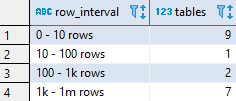## Number of tables by the number of rows in Vertica database

Article for:

Query below returns number of tables in predefined row count intervals. This allow you to get an overview of number of rows in your database.

## Query

``````with num_rows as (
select sc.schema_name,
p.anchor_table_name as table_name,
sum(sc.total_row_count - sc.deleted_row_count) as rows
from v_monitor.storage_containers sc
join v_catalog.projections p
on sc.projection_id = p.projection_id
and p.is_super_projection = true
group by schema_name,
table_name,
sc.projection_id
)
select row_interval,
count(*) as tables
from(
select t.table_schema,
t.table_name,
max(rows) as sort_col,
case when max(rows) > 1000000000 then '1b rows and more'
when max(rows) > 1000000 then '1m - 1b rows'
when max(rows) > 1000 then '1k - 1m rows'
when max(rows) > 100 then '100 - 1k rows'
when max(rows) > 10 then '10 - 100 rows'
else '0 - 10 rows' end as row_interval
from v_catalog.tables t
left join num_rows nr
on nr.schema_name = t.table_schema
and nr.table_name = t.table_name
group by t.table_schema,
t.table_name) itv
group by row_interval
order by max(sort_col);
``````

## Columns

• row_interval - predefined row count intervals:
• 0 - 10 rows
• 10 - 100 rows
• 100 - 1k rows
• 1k - 1m rows
• 1m - 1b rows
• 1b rows and more
• tables - number of tables whose row count falls in that interval

## Rows

• One row: number of tables in specific interval
• Scope of rows: all row count intervals that appear in the database
• Ordered by: from the smallest interval to the largest

## Sample results